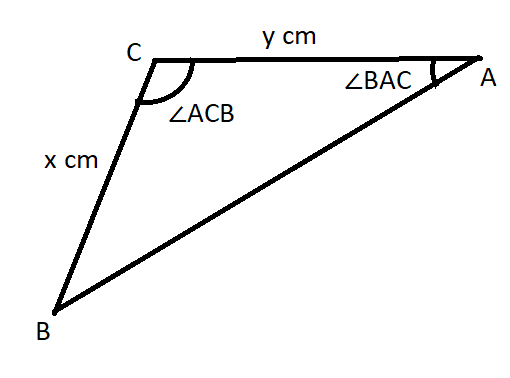# Consider the non-triangle below. Suppose that /_ACB=103^@ and m /_BAC=44^@, and that y=50.5cm. What of the value of X?01510101661.pngBevan Mcdonald 2021-01-16 Answered

Consider the non-triangle below. Suppose that $\mathrm{\angle }ACB={103}^{\circ }$ and $m\mathrm{\angle }BAC={44}^{\circ }$, and that $y=50.5cm$. What of the value of X?You can still ask an expert for help

• Questions are typically answered in as fast as 30 minutes

Solve your problem for the price of one coffee

• Math expert for every subject
• Pay only if we can solve itbrawnyN
We know that the sum of angles in a triangle equals 180 degrees. Therefore,
$\mathrm{\angle }BAC+\mathrm{\angle }cba+\mathrm{\angle }ACB={180}^{\circ }$
$\mathrm{\angle }CBA={18}^{\circ }-\mathrm{\angle }BAC-\mathrm{\angle }ACB$
$\mathrm{\angle }CBA={180}^{\circ }-{44}^{\circ }-{103}^{\circ }$
$\mathrm{\angle }CBA={33}^{\circ }$
By using law of sines in the given triangle,
$\left(\frac{\mathrm{sin}\mathrm{\angle }BAC}{x}\right)=\left(\frac{\mathrm{sin}\mathrm{\angle }CBA}{y}\right)$
$\left(\frac{{\mathrm{sin}44}^{\circ }}{x}\right)=\left(\frac{{\mathrm{sin}33}^{\circ }}{50.5}\right)$
$x=\frac{{\mathrm{sin}44}^{\circ }}{{\mathrm{sin}33}^{\circ }}\cdot 50.5$
$x=\frac{0.6946}{0.5446}\cdot 50.5$
$x=64.41$